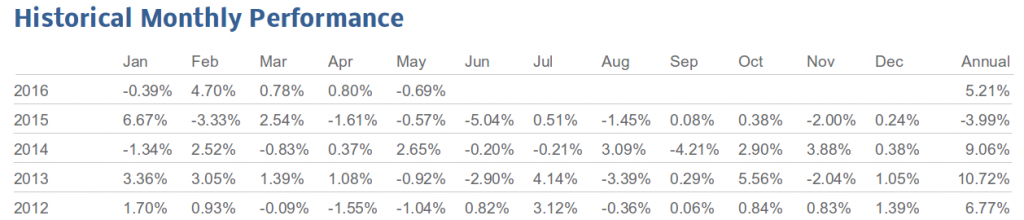A historical monthly performance table is used on almost every factsheet we produce for our customers. The example below shows the effective discrete performance of each month over a 5 year time horizon, with year-to-date (YTD) / annual performance.By looking at this table one would assume that all monthly performance numbers over one year add up to that year’s performance (in the last column). However, when taking a closer look at the example above you see that this is not the case. The effects of compounding returns over the course of a year are responsible for the differences. It is possible to calculate the YTD return using monthly returns, but the formula for doing so depends on the types of returns you are working with.

In the following post we provide a more detailed explanation on how to precisely calculate YTD performance using monthly or quarterly returns. In real life, discrete returns are pretty much always used when it comes to reporting performance figures. Thus, we will start with this case. However, the cases of linear as well as continuous returns will also be covered, for both completeness and to reinforce the concept.

For illustration purposes we will use the following price series as an example, with an initial price of 100 on the 31st of Dec 2014. We will look at quarterly returns (thus 4 periods in one year) for the sake of simplicity. The same is of course also true for monthly returns using 12 periods instead of 4.

Year 2015Price Series
End of Mar
101.00
End of Jun103.02
End of Sep106.1106
End of Dec105.049494

### Discrete Returns:

Given the end of quarter prices above, we can calculate discrete returns for the quarterly performance table from time perioduntilusing the following formula (see blog post on performance calculation), withthe given price at timeandthe given price at time:This results in:

CalculationDiscrete Returns
Mar101 / 100 - 11.00%
Jun103.02 / 101 - 12.00%
Sep106.1106 / 103.02 - 13.00%
Dec105.049494 / 106.1106 - 1-1.00%
YTD 2015105.049494 / 100 - 15.049494%

By calculating quarterly performance with this formula we are using the discrete paradigm for compounding interest rates. This means that interest rate payments are made at discrete points in time (at the end of each period) and are reinvested for the next time period.

Looking at 2015 prices we see different positive and negative discrete returns for single quarters. By simply adding up those numbers we compute a total performance of, but in fact YTD performance is 5.05% (rounded). This accurate discrete YTD performance of 5.05% (rounded) is also more intuitive since the price increased from 100 end of Dec 2014 to 105.049494 end of Dec 2015.

The assumption of summing up individual period returns to derive the correct aggregated multi-period performance return would only be correct in cases where the period returns were additive. This however is not the case with discrete returns (read more in our last blog post about additivity and multiplicativity of returns). Discrete returns are multiplicative, thus the correct aggregated performance is calculated using the following formula:Now let’s apply this formula to our example above. First we need to convert the performance numbers to decimals and add 1 to get the interest factor (return of 1.00% converts to the interest factor of 1.01). The next step is to calculate the product of the single interest factors:To get the YTD performance number we have to subtract 1:The formula to aggregate single period returns to an overall multi-period return of course does not assume quarterly periods so it can also be used for any other period schema like e.g. monthly returns.

As written in the beginning of this article we want to show how to handle aggregating single-period returns in the cases of linear returns and continuous returns. For further information on those cases you can find more details below.

### Linear Returns:

Linear returns in a multi-period setting are calculated using the following formula:Thus we get for our example:

CalculationLinear Returns
Mar(101 - 100) / 1001.00%
Jun(103.02 - 101) / 1002.02%
Sep(106.1106 - 103.02) / 1003.0906%
Dec(105.049494 - 106.1106) / 100-1.061106%
YTD 2015(105.049494 - 100) / 1005.049494%

We see that the quarterly linear return numbers are slightly different compared to the quarterly discrete return numbers. Summing up those single-period linear returns results infor the aggregated multi-period performance which equals the accurate YTD performance of 5.05% (rounded). So by adding up the single-period linear returns we can actually compute the overall multi-period performance as linear returns are additive.

Note: When calculating with discrete returns we get the same YTD performance figure as the formulas for calculating single-period returns for linear as well as discrete returns are the same.

### Continuous Returns:

Finally we use the same price series from the above example to calculate the effective continuous performance numbers. Given the pricesat timeandat timethe continuous returns for the time perioduntilcan be calculated using the following formula:Thus we get:

CalculationContinuous Returns
Marln(101 / 100)0.9950330853%
Junln(103.02 / 101)1.98026273%
Sepln(106.1106 / 103.02)2.955880224%
Decln(105.049494 / 106.1106)-1.005033585%
YTD 2015ln(105.049494 / 100)4.926142454%

We see again that the quarterly return numbers are slightly different compared to the discrete and linear returns. As continuous returns are additive, like linear returns, the single-period continuous returns can be summed up to get the overall multi-period return of 4.93% (rounded):### Conclusion:

As you can see, although the prices of the financial asset we look at are the same, thus asset growth being the same, the quarterly as well as the overall YTD returns are different depending on the compounding model used. The choice of the compounding model does not effect wealth but it affects the actual performance figures as well as the calculation rules which you use to e.g. aggregate single-period returns to an overall multi-period performance.

WEBINAR